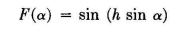# Properties of the horizontal dipole above the ground

The radiation pattern in the vertical plane perpendicular to a horizontal dipole over perfectly conducting ground is given by the equation:where α is the angle to the horizon (the radiation characteristics in the facade perpendicular to the axis of the dipole), and h is the height above ground in electrical degrees (where 360 electrical degrees correspond to one wavelength).

The values of a at which nulls and maximums occur can be found by equating this to 0 and 1.0, respectively. For heights greater than 180 degrees (that is, a h height greater than ½ of the wavelength) there will be multiple nulls and maximums, the number increasing with the height h.Vertical patterns from this equation are given in Fig. 1, and a chart giving the angles of nulls and maximums is given in Fig. 2 (where the horizontal axis is expressed in electrical degrees).

In most cases these patterns are used for typical imperfectly conducting grounds such as are encountered in practice. However, to compute the precise pattern that will result from the antenna over ground of the type present at a specified location, its conductivity a and inductivity e and the frequency / of operation must be determined.

The effect of ground loss on the maximum field strength, in comparison with perfectly conducting ground (σ = ∞) giving zero ground loss, is shown in Fig. 3. These data are approximate but show the importance of ground loss for small heights.

This equation influences the pattern primarily with respect to the decrease in the amplitude of the maximums and the slight filling of the nulls due to incomplete cancellation of direct and reflected fields, as compared with perfectly reflecting ground. The angles of maximums and minimums are only slightly affected and for most practical uses may be considered to be the same.